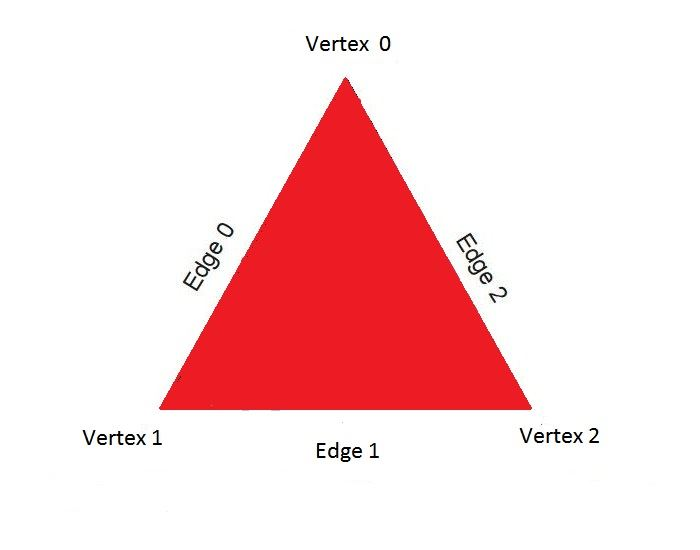# Triangle Worksheet

Most of us would be aware of the fact that triangles would be an important topic while studying maths in school. A triangle is no more than a polygon with three vertices and three edges and an integral part of geometry being it as one of the basic shapes. Triangles are assumed to be figures that have two dimensions except in some rare cases.

Here we are providing Triangle Math worksheets that comprise the identification and recognition of triangles by criteria like area and perimeter, interior and exterior angles, sides and angles, simple algebra problems area for typical triangles like scalene and isosceles and equilateral ones and so on.Let us take an example of the isosceles triangle which is classified as a one by length, and it has sides having an equal length. Notably, the opposite sides have their angle measure being similar owing to the backing of the isosceles angle theorem. There are different Math formulas for finding the area of a triangle.

Another example of triangles based on their internal angles measured regarding degrees would be that of a right-angled triangle. This triangle is called as a right-angled owing to one of its angles measuring 90 degrees. The longest side of the triangle placed opposite the right angle is called the hypotenuse, and the other sides are its altitude and base respectively with the former being at the top of the right angle and the latter at the bottom of the right angle. The right-angled triangles are renowned for their connection to the Pythagoras Theorem. The theorem states that the sum of the squares of the altitude and base would be equal to the square length of the hypotenuse and are studied by students of mathematics globally.

The above examples of a triangle would have given you an overview of the significance of triangles in the mathematical branch of geometry.

## Triangles Worksheet for Class 9

1. Find the measure of an angle which is 36° more than its complement.
2. Find the measure of an angle which is 25° less than its supplement.
3. Two supplementary angles are in the ratio 3:2. Find the angles.
4. Two lines AB and CD intersect at O. If ∠AOC=50°, find ∠AOC, ∠BOD and ∠BOC
5. The vertical angle of an isosceles triangle is 100°. Find its base angles.

Triangles worksheet PDF for class 9 to solve more problems:-Download PDF Here

## Triangles Worksheet for Class 10

1. The areas of two similar triangles are 81 cm² and 49 cm² respectively. Find the ratio of their corresponding heights. What is the ratio of their corresponding medians?
2. The areas of two similar triangles are 169 cm 2 and 121 cm respectively. If the longest side of the larger triangle is 26 cm, find the longest side of the smaller triangle.
3. The corresponding altitudes of two similar triangles are 6 cm and 9 cm respectively. Find the ratio of their areas.
4. ABC is a triangle in which ∠ A = 90°, AN ⊥ BC, BC = 12 cm and AC = 5 cm. Find the ratio of the areas of ΔANC and ΔABC.
5. The corresponding altitudes of two similar triangles are 6 cm and 9 cm respectively. Find the ratio of their areas.

Triangles worksheet PDF for class 10 to solve more problems:-Download PDF Here

### Video Lesson on Triangles